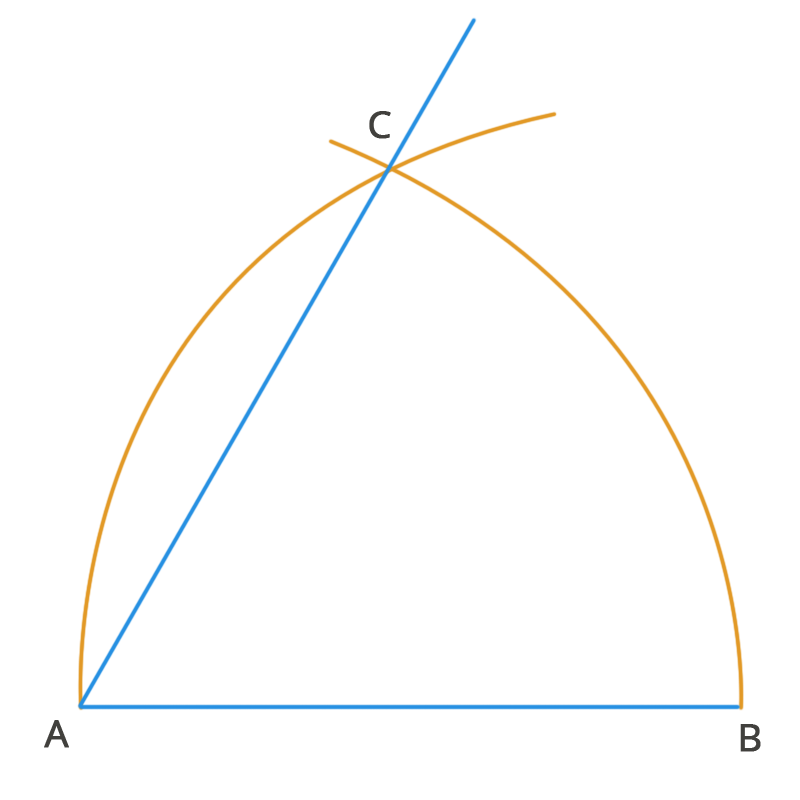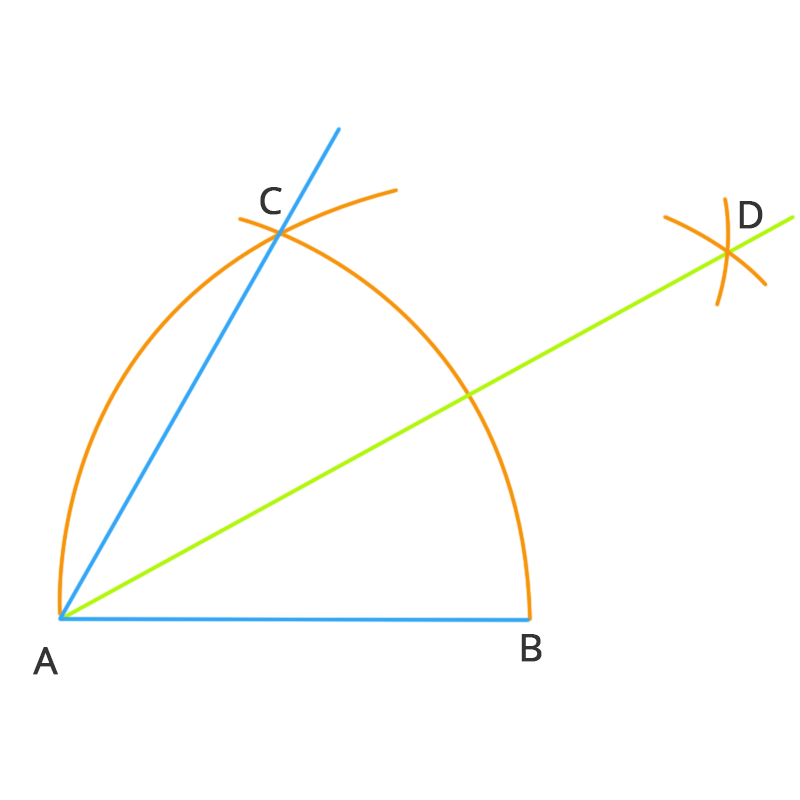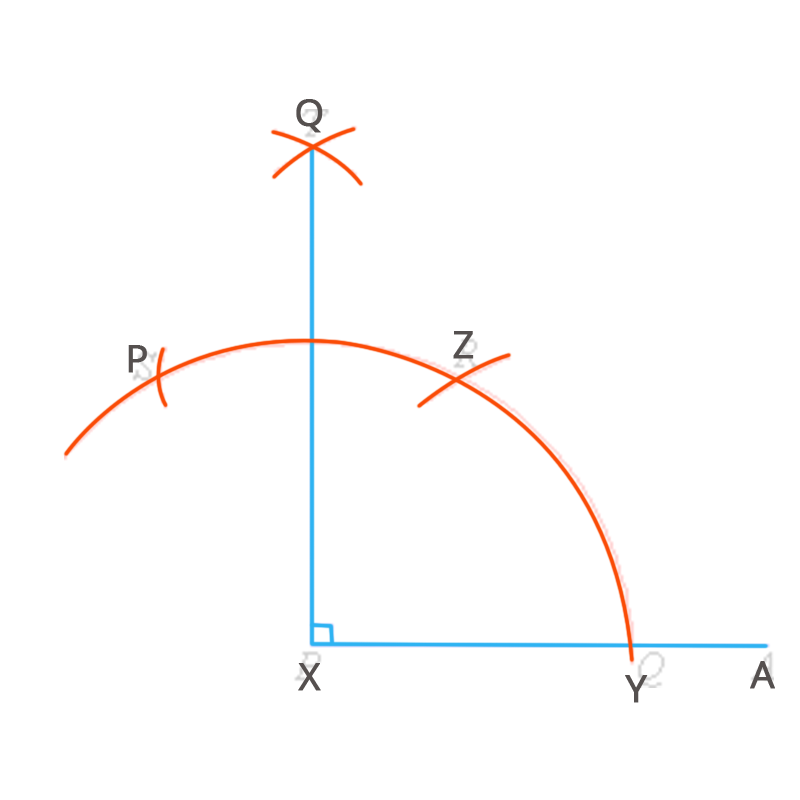• Call Now

1800-102-2727•

# Construction of Angles

Geometry is a major topic in mathematics where one can study different angles, shapes, sizes etc. In geometry, the construction of angles plays a very important role, as it serves as a basis for all the other concepts related to angles and shapes. The following are the unique steps given in order to construct shapes using different angles;## 1. Construction of a 60 angle

In equilateral triangles, all angles form 60 to each other. Following are the steps to construct a 60 angled triangle;
Step 1 – Draw a line segment AB.
Step 2 – Now, place the compass at A and draw an arc so that it passes through B.
Step 3 – Then, place the compass point at B and draw another arc that passes through A. The
arc created in this step should have to cut the arc previously drawn in the above step.
Step 4 – Name the new point as C and then join A and C.
Step 5 – This measures 60 while an equilateral triangle ABC is formed.## 2. Construction of a 30 angle

It is commonly known that 30 is the bisector of 60. So, in order to create a 30 angle, we can simply apply this common knowledge. Following are the steps involved:
Step 1 – Before constructing the angle of 30, we need to first create an angle of 60 and then bisect it further.
Step 2 – Draw an arm AB, then place the compass at A and draw an arc that passes through B.
Step 3 – Locate the compass point at B, followed by drawing an arc that cuts the previously drawn arc at a new point C.
Step 4 – Now, while placing the compass at C, keeping the compass radius the same, draw another arc near the arc containing the point C.
Step 5 – Next, extend the arc of C where it cuts the old arc drawn in step 4 at D.
Step 6 – Now join the points D and A. Finally, the angle BAD is 30.## 3. Construction of a 90 angle

When two lines make 90° to each other, they can be considered normal lines or perpendicular lines. They are also called right-angled triangles. To construct a 90there are two common ways. One is by simply bisecting a straight line, and the other one is mentioned below,
Step 1 – Firstly, draw the arm XA.
Step 2 – Then, place the sharp point of the compass at X and draw a small arc that cuts the arm at Y.
Step 3 – Now, place the compass point at Y, drawing an arc of more or less the same radius as XY. The currently drawn arc must cut the previously drawn arc in the above step at a new point called Z.
Step 4 – Now locate the compass at the point Z and then draw another arc containing the same radius as XY to cut the arc drawn during step 2 at another point and name it as P.
Step 5 – With the compass at Z, extend the arc containing P slightly longer.
Step 6 – Now, while placing the point at P, draw another arc of the same radius as XY and cut the previously drawn arc at step 5 at Q.
Step 7 – Finally, join the point Q to X with the help of a ruler. The angle AXQ forms 90.

Hence these are some of the common angles that can be constructed easily with the help of a geometric tool called a compass.Talk to our expert
Resend OTP Timer =
By submitting up, I agree to receive all the Whatsapp communication on my registered number and Aakash terms and conditions and privacy policy